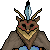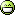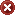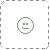Deviant Art Shop Forum More Submit Join LoginThe Mathematical Domains I by

Thanks to Alpkatchen ("there is always more") are known the existence of extremely complex and numerous divisions of the Reality very far from the common idea of Planar Spaces.

Because Sercque can penetrate (albeit briefly) in the ∞ ρ-Milegu, they got into contact with species that, even without the powers individually like the Lttbeh Races, they knew very complex levels of Reality, stratum that other races can reach and that the Lttbeh Reaces themselves didn't have explored before.

They contacted the Noiasanaga, inhabitants of "It Abstract", one of the deep Alpkatchen domains. Thanks to this strange species, Sercque found out the existence of "Fragmented Abstract Worlds" (also called by Noiasanaga as "Abstract Fragments") and Mathematical Domains.

While Abstract Fragments were terribly complex to define at 100% (is inherent in them remain undefined) Mathematical Domains proved to be an outstanding focus of study.

Mathematical Domains are "Planar Spaces" of abstract type (not all make themselves Spaces) defined by mathematical operations that emerge from each event, interaction or place in Milegu.

That is, everything that "is" (and everything that "is not") causes, by their very existence (and non-existence), a series of mathematical operations highly diverse (ie, intervenes the is-doesn't-exist). Attempts to define concretely operations and mathematical objects produced by the Reality have often been unsuccessful.

One of the best attempts to understand the enormous complexity of Mathematical Domains was carried by Cxȱ'qre, who developed an amazing science to describe these operations emerging from Reality as such operations and mathematical interactions caused the emergence of new Mathematical Domains.

## Relationship of the Mathematical Domains with Essences

By its defining character of the Realities of the Milegu they are (the Mathematical Domains) rightly compared to Milegu Essences. One of the key differences of Mathematical Domains is that the information that is stored by them it is not stored in a discrete basis (as they do the Essences), although neither Domains Mathematical Essences or have a definable physical entity without using concepts Annexes Milegu (complex properties than the Space, Time, Geometry, elemental forces, etc. such as Njiruh, the Qnch'ur'u, the Anūbuḫu, or the Namosë), but have a continuum character (and may even form create their own Spaces inside the Mathematical Domains).

This difference is most easily understood when we compare an Essence of a sword with the Mathematical Domain defines this sword.

First, the Essence of a sword is a metaphysical object of discrete nature that contains all the information that describes that sword. However, the Mathematical Domain of that sword is not defined directly, since Mathematical Domains not describe Reality discretely but continuously: Mathematical Domains "of the sword" includes not only the sword itself but more extensive, encompassing everything around 1,2.

Second, the Essences of Milegu and Mathematical Domains have another fundamental difference: while "Abstract Information" of the objects found in the essences of "pure" way, in Mathematical Domains is described purely mathematically: defining all possible information of an Essence, but moved to operations and exotic mathematical objects.

The relationship between Milegu Essences and Mathematical Domains is so close that many scholars species about Essences, such as Ilyumë of Echlye, consider to Mathematical Domains as a special type of Essence which encompasses an entire Reality (is the sum of all Essences of that Reality) and displayed continuously. Ie, (parts of) Mathematical Domains could become Essences and vice versa.

## Relationship to Other Planar Structures

The relationship of Mathematical Domains with other Planar Structures is evident. First, their permanent self-definition of all Milegu a whole makes the Mathematical Domains a self-contained (and equal) entity to the Figure most perfect of All (which would be one of its many aspects, although Mathematical Domains also define each one of the other aspects of the Figure). Because being a self-contained structure Planar generate (by itself) Windows of Content: ie finite points where are huge portions of Milegu.

It is not only related to Mathematical Domains or with the Figure most perfect of All but also with all other Final Structures as Ealqum: Mathematical Domains also be an immeasurable source of information for the growth of the Figure most perfect of All 3.

For its defining character of Realities and also its mathematical character, Mathematical Domains have been compared with the Statistic-Planes, which store information for specific events differentiated from each other, making beings who visit the Statistic-Planes can accurately quantify anything. This information, to be totally accurate, it is valuable for all types of activities, from science to warfare.

However, the big problem of the Statistic-Planes is that being part of the same figure, are very difficult to access .

So, it have been implemented methods on Mathematical Domains to find there the same information that appears in the Statistic-Planes (with mixed results). The slow pace is so extreme that it is almost impossible to extract information from the Mathematical Domains, as well as being surrounded by a very high information noise 4.

The property of the Mathematical Domains of describe any situation, object or place (including those belonging to higher levels of complexity —the Depths of Alpkatchen—, make many scholars consider the possibility that the whole Milegu can be computable, represented total way Computational Planes or by a Single computation Plane.

Analyzing other Planar Structures such as the Logical-mathematical borders, we would find that they are a simple superposition of Mathematical Domains that emerge and can be analyzed in the boundaries between Realities .

*1: I mean, the Mathematical Domain of a sword that is found in a particular Reality (Planar Space, Dimension, Plane, Shain, etc. ) encompasses all that Reality.
*2: The Essence of Milegu also include in their description to objects in the environment, but only in relation to the object itself and the Essence itself.
*3: Here is related to 0-Milegu and not ∞p-Milegu. In the 0-Milegu, the Figure, despite being infinite, can grow.
*4: The own search within the Mathematical Domains generates new information that hinders the search.Featured By Owner Jan 22, 2016  Hobbyist Writer
A lot of relations and interconections.Add a Comment: